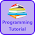# Programming Videos

## Search

### Program to Find Smallest of three Numbers in C, C++Program to Find Smallest of Three Numbers
Write a C, C++ program to find smallest of three numbers. Given an input three numbers, We have to write a code to find smallest of three numbers.

In this question, We take three input numbers from a user and print the smallest of three numbers.

Find largest of three numbers

Find smallest number without using comparison operator

Program to find smallest number of an array

Algorithm to Find Smallest of three Numbers

1. Declare three variable a ,b, c.

2.  Compare a with b and c. If a is smaller than b and c than a is smallest among three numbers.

3. Compare b with a and c. if b is smaller than a and c than b is smallest among three numbers.

4. Else c is smallest among three numbers.

## Find Smallest of three Numbers in C++

```#include <iostream>
using namespace std;

int main() {

int a, b, c;

cout << "Enter three numbers \n";

/* Taking input */
cin >> a >> b >> c;

/* If a is smaller than b and c. */

if (a < b && a < c) {
cout << "Smallest number is " << a;

/* If b is smaller than a and c */
} else if (b < a && b < c)  {
cout << "Smallest number is " << b;

} else {
cout << "Smallest number is "<< c;

}

return 0;
}

```

Output :

Enter three numbers :  8       1       5

Smallest number is : 1

## Find Smallest of three Numbers in C

```#include <stdio.h>

int main(void) {

int a, b, c;

printf("Enter three numbers \n");
scanf("%d %d %d", &a , &b , &c);

if (a < b && a < c) {
printf("Smallest number is %d " ,a);

} else if (b < a && b < c) {
printf("Smallest number is %d " ,b);

} else {
printf("Smallest number is %d ", c);

}

return 0;
}

```

Similar Questions

Program to find smallest number in an array.

1.What if I enter all three as same number.
It should write smallest of three as the same number. Isn't it?

1.Yes, good catch.

2.What if I want the program to find the smallest number from 6 numbers?

3.In that case try to use array. Here is a sample program for that

http://www.cprogrammingcode.com/2014/03/write-program-to-find-smallest-element.html